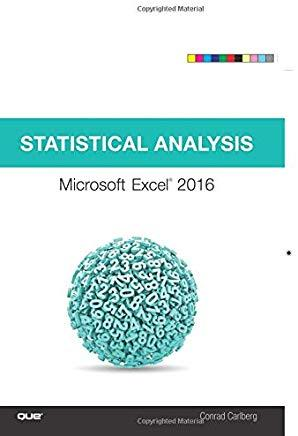### Statistical Analysis: Microsoft Excel 2016### Huge savings for students

Each student receives a 50% discount off of most books in the HSG Book Store. During class, please ask the instructor about purchase details.
 List Price: \$44.99 Price: \$22.50 You Save: \$22.50
4USE EXCEL'S STATISTICAL TOOLS TO TRANSFORM YOUR DATA INTO KNOWLEDGE

Nationally recognized Excel expert Conrad Carlberg shows you how to use Excel 2016 to perform core statistical tasks every business professional, student, and researcher should master. Using real-world examples and downloadable workbooks, Carlberg helps you choose the right technique for each problem and get the most out of Excel's statistical features. Along the way, he clarifies confusing statistical terminology and helps you avoid common mistakes.

You'll learn how to use correlation and regression, analyze variance and covariance, and test statistical hypotheses using the normal, binomial, t, and F distributions. To help you make accurate inferences based on samples from a population, Carlberg offers insightful coverage of crucial topics ranging from experimental design to the statistical power of F tests. Updated for Excel 2016, this guide covers both modern consistency functions and legacy compatibility functions.

Becoming an expert with Excel statistics has never been easier In this book, you'll find crystal-clear instructions, insider insights, and complete step-by-step guidance.

• Master Excel's most useful descriptive and inferential statistical tools
• Understand how values cluster together or disperse, and how variables move or classify jointly
• Tell the truth with statistics--and recognize when others don't
• Infer a population's characteristics from a sample's frequency distribution
• Explore correlation and regression to learn how variables move in tandem
• Use Excel consistency functions such as STDEV.S( ) and STDEV.P( )
• Test differences between two means using z tests, t tests, and Excel's Data Analysis Add-in
• Identify skewed distributions using Excel's new built-in box-and-whisker plots and histograms
• Evaluate statistical power and control risk
• Explore how randomized block and split plot designs alter the derivation of F-ratios
• Use coded multiple regression analysis to perform ANOVA with unbalanced factorial designs
• Analyze covariance with ANCOVA, and properly use multiple covariance
• Take advantage of Recommended PivotTables, Quick Analysis, and other Excel 2016 shortcuts
Que